# More Than / Less Than Worksheets

## More or less?  The ordering of numbers

These printable worksheets help kids learn concepts related to more, less, greatest, least and the ordering of numbers.  We also introduce the use of more than / less than / equal to symbols (>, <, =).

## Circle the group with more (fewer) objects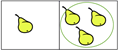Our more / fewer worksheets simply ask students to identify which group has more, or fewer, objects.  No numbers are used.

## Draw more (or less) objects than shown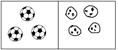In these draw more or less objects worksheets students draw a group of objects with more (or less) objects than the one shown.  No numbers are used.

## Circle the group with the most objects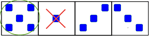Students select the groups with the most and least objects in these most - least worksheets.  No numbers are used so as to emphasize the underlying meaning of "most" and "least" .

## Count the objects and circle the correct symbol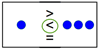These  more than - less than symbols worksheets introduce students to the more / less / equal symbols in the context of groups of objects rather than numbers.

## Circle the number which is greater (less)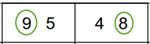These greater than - less than worksheets ask students which number (as opposed to a group of objects) is greater than or less than the other.

## Compare the numbers and write in the correct symbol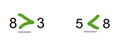These comparing numbers worksheets ask students to use the more than less than symbols to compare numbers (rather than groups of objects).

## Circle the greatest (least) number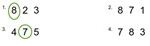Students circle the greatest or least number in these greatest-least worksheets.

## Order the numbers from least to greatest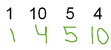These ordering numbers worksheets ask students to re-order 4 numbers  from least to greatest.

## More numbers worksheets

Explore all of our learning numbers worksheets (recognizing and printing numbers), counting worksheets (counting objects, skip counting, counting backwards) and comparing numbers worksheets ("more than", "less than", ordering numbers).

## What is K5?

K5 Learning offers reading and math worksheets, workbooks and an online reading and math program for kids in kindergarten to grade 5.  We help your children build good study habits and excel in school.